Custom SearchPRACTICE PROBLEMS: Differentiate the functions in problems 1 through 3.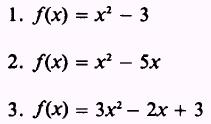4. Find the slope of the tangent line to the curve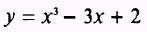at the points x = - 2, 0, and 3 5. Find the values of x where the function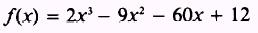has a maximum or a minimum. ANSWERS: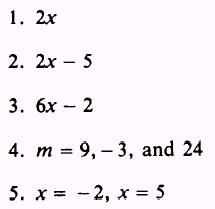SUMMARY The following are the major topics covered in this chapter: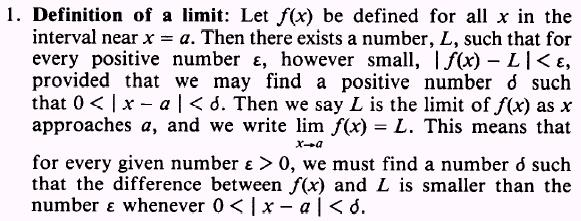2. Indeterminate forms: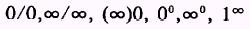Two methods of evaluating indeterminate forms are (1) factoring and (2) division of the numerator and denominator by powers of the variable. 3. Limit theorems: Theorem 1. The limit of the sum of two functions is equal to the sum of the limits: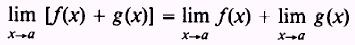This theorem can be extended to include any number of functions, such as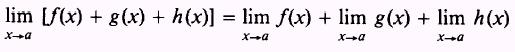Theorem 2. The limit of a constant, c, times a function, fx), is equal to the constant, c, times the limit of the function: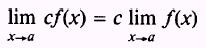Theorem 3. The limit of the product of two functions is equal to the product of their limits: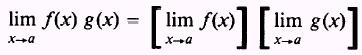Theorem 4. The limit of the quotient of two functions is equal to the quotient of their limits, provided the limit of the divisor is not equal to zero:4. Infinitesimals: A variable that approaches 0 as a limit is called an infinitesimal:The difference between a variable and its limit is an infinitesimal: If lim V = L, then lim V - L = 0 5. Sum and product of infinitesimals: Theorem 1. The algebraic sum of any number of infinitesimals is an infinitesimal. Theorem 2. The product of any number of infinitesimals is an infinitesimal. Theorem 3. The product of a constant and an infinitesimal is an infinitesimal. 6. Continuity: A function, f(x), is continuous at x = a if the following three conditions are met:7. Discontinuity: If a function is not continuous at x = a, then it is said to be discontinuous at x = a. 8. Ways of expressing the derivative of y with respect to x:9. Increment method for obtaining the derivative of y with respect to x: 1. Set up the function of x as a function of (x +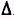x) and expand this function. 2. Subtract the original function of x from the new function of (x +x). 3. Divide both sides of the equation byx. 4. Take the limit of all the terms in the equation asx approaches zero. The resulting equation is the derivative of f(x) with respect to x. General formula for the derivative of any expression in x: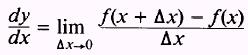11. Maximum or minimum points on a curve: Set the derivative of the function, f(x), equal to zero and determine the values of the independent variable that will make the derivative equal to zero. (Note: When the derivative of a function is set equal to zero, that does not mean in all cases the curve will have a maximum or minimum point.) ADDITIONAL PRACTICE PROBLEMS Find the limit of each of the following: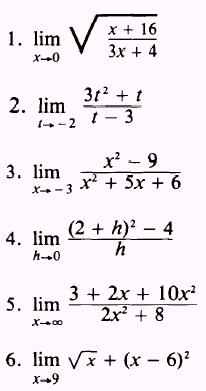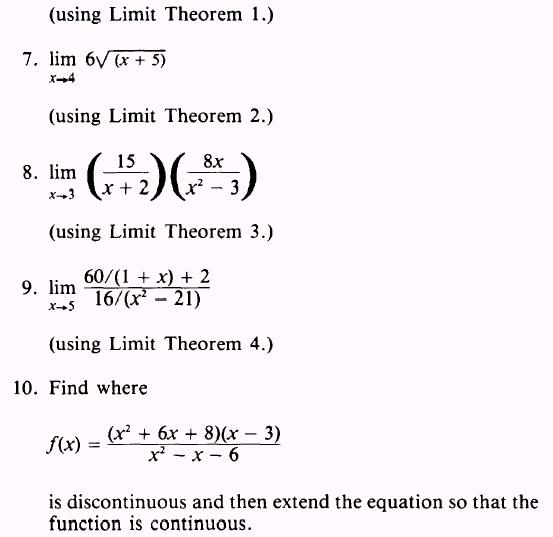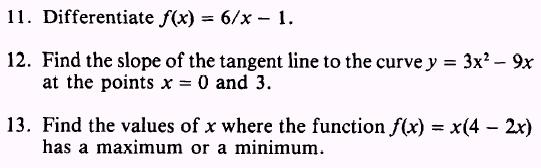ANSWERS TO ADDITIONAL PRACTICE PROBLEMS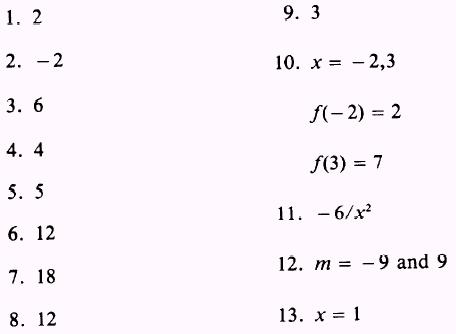Integrated Publishing, Inc. - A (SDVOSB) Service Disabled Veteran Owned Small Business